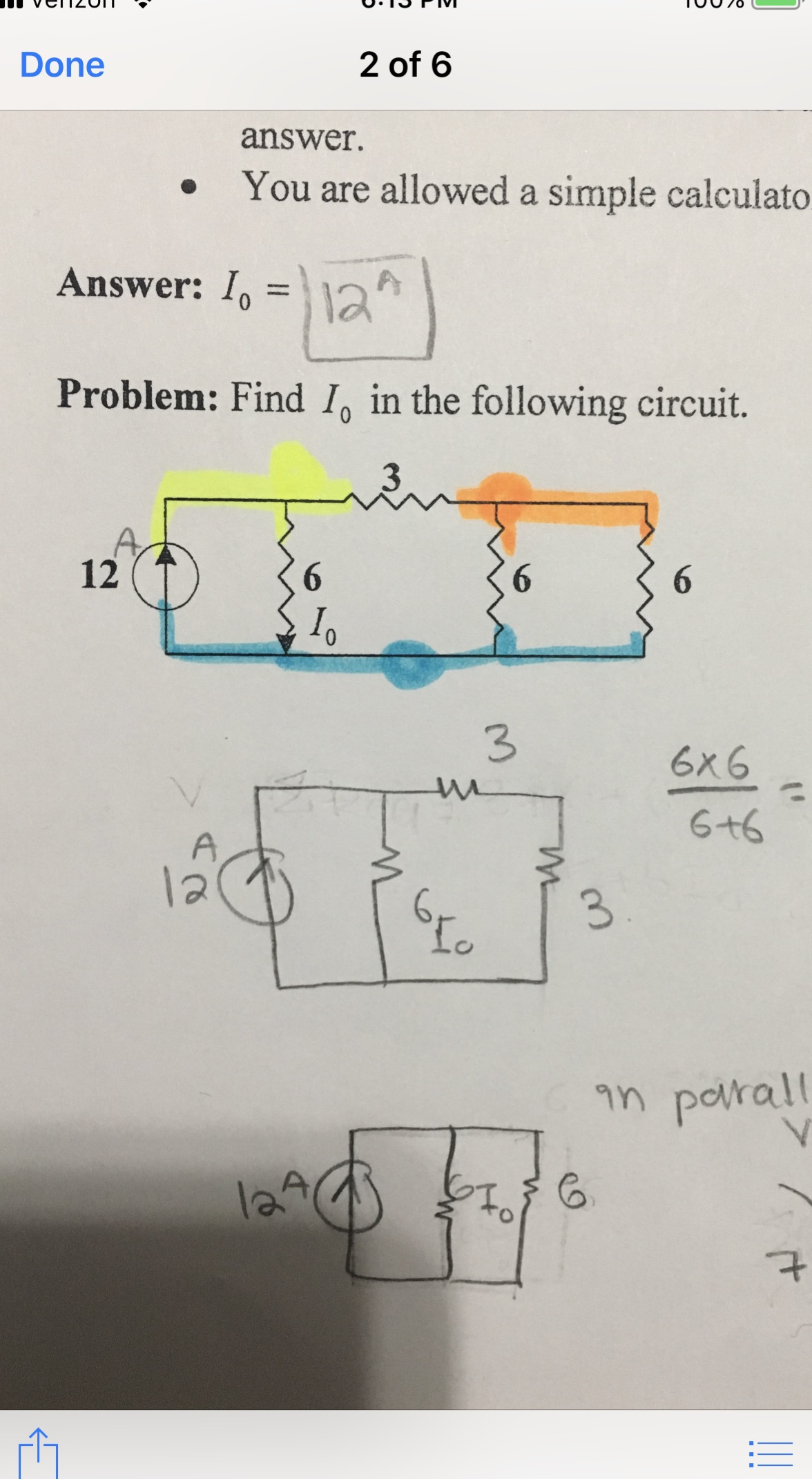Done2 of 6answer.You are allowed a simple calculatoAnswer:AProblem: Find Io in the following circuit.336x66+6laLoin porallle

Questionhelp_outlineImage TranscriptioncloseDone 2 of 6 answer. You are allowed a simple calculato Answer: A Problem: Find Io in the following circuit. 3 3 6x6 6+6 la Lo in porall le fullscreen
Step 1

The circuit Diagram is shown on the board:

At node B, the two 6Ω resistors connected are in parallel first we solve this parallel circuit

Step 2

Consider the output resistance to be R1 whose calculation is shown on the board:

Now the 3Ω resistance connected at node A will be in series with the resistance R1...

Want to see the full answer?

See Solution

Want to see this answer and more?

Our solutions are written by experts, many with advanced degrees, and available 24/7

See Solution
Tagged in

Electrical Engineering